# Action of a group on a manifold

(diff) ← Older revision | Latest revision (diff) | Newer revision → (diff)

The best-studied case of the general concept of the action of a group on a space. A topological groupacts on a spaceif to eachthere corresponds a homeomorphism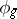of(onto itself) satisfying the following conditions: 1)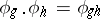; 2) for the unit element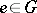the mapping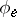is the identity homeomorphism; and 3) the mapping,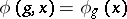is continuous. Ifandhave supplementary structures, the actions ofwhich are compatible with such structures are of special interest; thus, ifis a differentiable manifold andis a Lie group, the mappingis usually assumed to be differentiable.

The set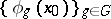is called the orbit (trajectory) of the point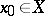with respect to the group; the orbit space is denoted by, and is also called the quotient space of the spacewith respect to the group. An important example is the case whenis a Lie group andis a subgroup; thenis the corresponding homogeneous space. Classical examples include the spheres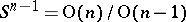, the Grassmann manifolds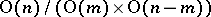, and the Stiefel manifolds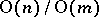(cf. Grassmann manifold; Stiefel manifold). Here, the orbit space is a manifold. This is usually not the case if the action of the group is not free, e.g. if the setof fixed points is non-empty. A free action of a group is an action for whichfollows if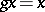for any. On the contrary,is a manifold ifis a differentiable manifold and the action ofis differentiable; this statement is valid for cohomology manifolds overfor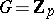as well (Smith's theorem).

Ifis a non-compact group, the space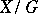is usually inseparable, and this is why a study of individual trajectories and their mutual locations is of interest. The group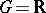of real numbers acting on a differentiable manifoldin a differentiable manner is a classical example. The study of such dynamical systems, which in terms of local coordinates is equivalent to the study of systems of ordinary differential equations, usually involves analytical methods.

Ifis a compact group, it is known that ifis a manifold and if each,, acts non-trivially on(i.e. not according to the law), thenis a Lie group . Accordingly, the main interest in the action of a compact group is the action of a Lie group.

Letbe a compact Lie group and letbe a compact cohomology manifold. The following results are typical. A finite number of orbit types exists in, and the neighbourhoods of an orbit look like a direct product (the slice theorem); the relations between the cohomology structures of the spaces,and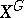are of interest.

Ifis a compact Lie group,a differentiable manifold and if the action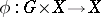is differentiable, then one naturally obtains the following equivalence relation:if and only if it is possible to find an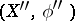such that the boundary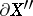has the formand such that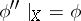,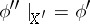. If the groupacts freely, the equivalence classes can be found from the one-to-one correspondence with the bordisms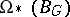of the classifying space(cf. Bordism).

Recent results (mid-1970s) mostly concern: 1) the determination of types of orbits with various supplementary assumptions concerning the groupand the manifold(); 2) the classification of group actions; and 3) finding connections between global invariants of the manifoldand local properties of the group actions ofin a neighbourhood of fixed points of. In solving these problems an important part is played by: methods of modern differential topology (e.g. surgery methods);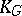-theory , which is the analogue of-theory for-vector bundles; bordism and cobordism theories ; and analytical methods of studying the action of the groupbased on the study of pseudo-differential operators in-bundles , .

How to Cite This Entry:
Action of a group on a manifold. Encyclopedia of Mathematics. URL: http://encyclopediaofmath.org/index.php?title=Action_of_a_group_on_a_manifold&oldid=14980
This article was adapted from an original article by A.V. Zarelua (originator), which appeared in Encyclopedia of Mathematics - ISBN 1402006098. See original article